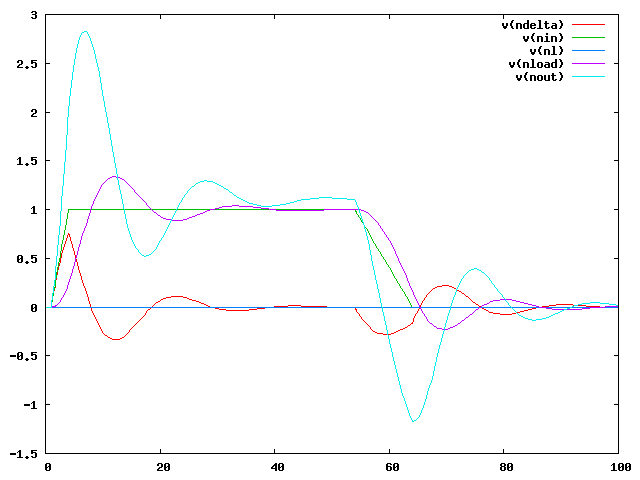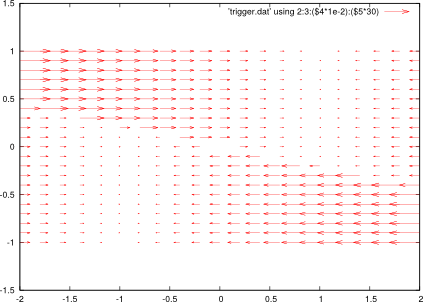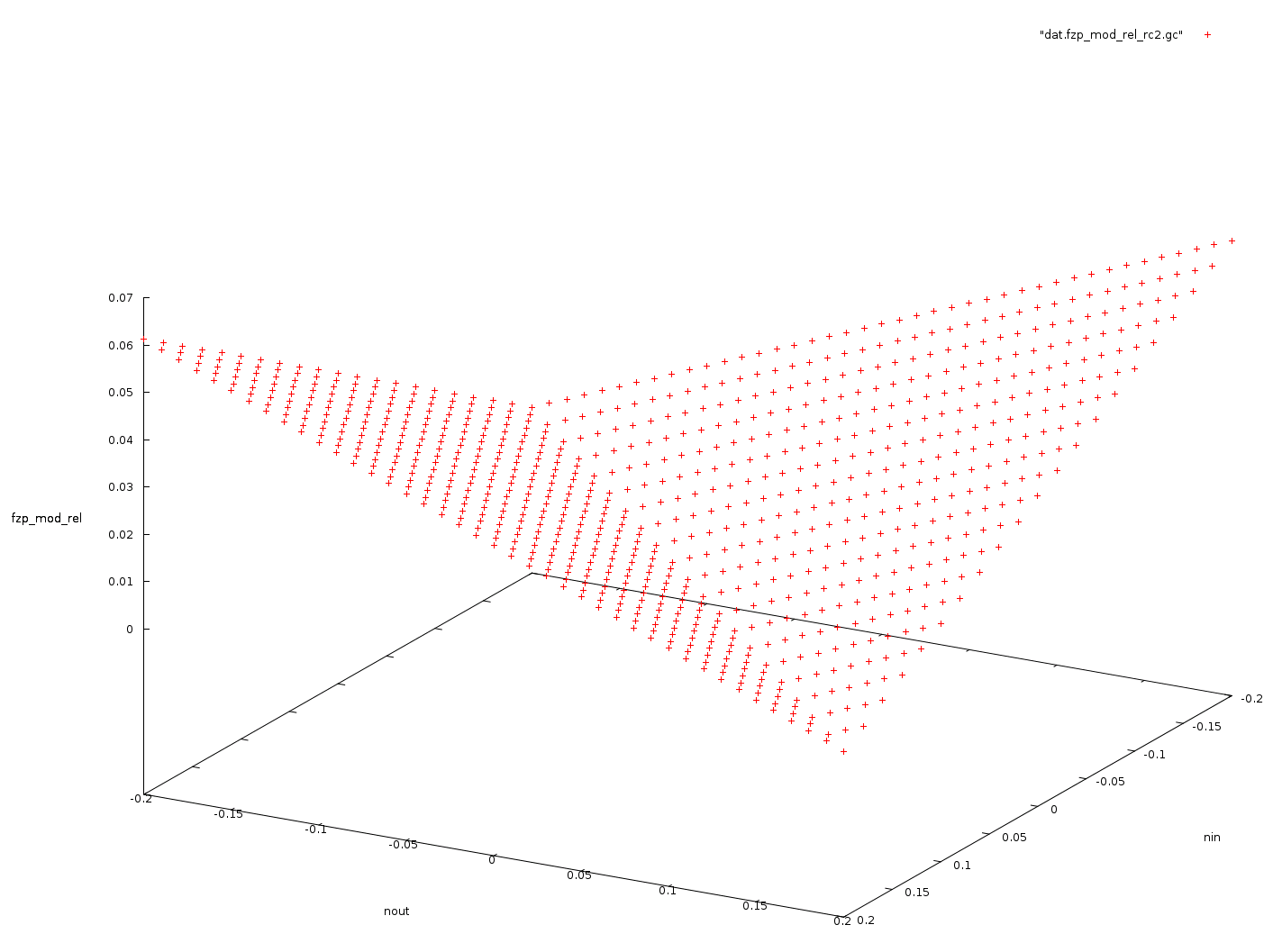# Vera / Gnucap UF

Vera is an equivalence checking tool for nonlinear analog circuits. Gnucap-UF is a gnucap fork targeted at research and experiments. The following tarballs are available: gnucap-uf, vera-static-binary and gnucap-adms-plugin. See here for instructions on how to build debian binary packages.

## Verilog-A Code Generator

The gnucap-adms package provides an admsXml based model compiler for devices written in verilog-a. It covers a (growing) subset of verilog-a. To get a loadable module from verilog-a, (install gnucap-adms and) type gnucap-adms -c device.va. Sometimes it is more convenient to embed models into netlists. See the following example.

```load lang_adms.so

options reltol=1e-4

`include "discipline.h"

module pid(sp,sn,cp,cn);
in sp,sn,cp,cn;
electrical sp,sn,cp,cn;

parameter real p = 1 from [0:inf);
parameter real i = 1 from [0:inf);
parameter real d = 1 from [0:inf);

analog begin
V(sp,sn) <+ p * V(cp,cn);
V(sp,sn) <+ i * idt(V(cp,cn));
V(sp,sn) <+ d * ddt(V(cp,cn));
end
endmodule

paramset mypid pid;
.p=1;
.i=1;
.d=.05;
endparamset

mypid pid1 (nout, 0, ndelta, 0);
spice

E1 ndelta 0 nin nload 1

rfake0 nin ndelta 1e12

Vreg nin 0 pulse iv=0 pv=1 rise=3 delay=1 width=50 fall=10

ll 0 nl 10u

.print tran v(nodes) method(pid1)
.tran 0 100 100 trace=a > pid.out echo

.options outwidth 200
.tran 0 100 1 trace=n

.print ddc v(nodes) dv(nodes)
.ddc
.end
```## State Space Inspection

Dynamic dc (ddc) analysis analyzes the dynamics of consistent operating points. For example, consider a simple schmitt trigger circuit and its operating points parameterized by input voltage and capacitor charge. ddc analysis computes the time derivatives of the node voltages (dv) at a consistent operating point close to the specified initial conditions.

```spice

.param a=.5
.param q1=0

v1 nin 0 pulse iv={-a} pv=a rise=1 period=2 fall=1 delay=0 width=0

.subckt trigger nin nout vss gnd vdd
E1 ne gnd np gnd tanh 1k 1
re ne nout 100
R1 np nin 1k
r2 np nout 5k
C1 nout gnd 1m ic=q1
* C2 np gnd 10n
.ends

Xt nin nout 0 0 0 trigger

.print ddc v(Xt.c1) v(v1) dv(nout) dv(nin)
.ddc q1 -2 2 .2 a -1 1 .1 > trigger.dat echo

.end
```
The output file trigger.dat contains the vector flow on the state space. The operating points are the zeroes of the vector field. The two connected components of the two contractive zeroes are clearly visible in the plot. These are the stable states of the circuit.## Formal Verification (Equivalence Checking)

Our verification tool Vera uses gnucap-uf as a simulator backend. It is available as a binary executable (here) ready to use. Consider two similar lowpass filters.

and

The equivalence check yields a measure for the difference of these filters. For example, we may plot the difference in dynamic behaviour as a function over the state space.